Refer to our Texas Go Math Grade 6 Answer Key Pdf to score good marks in the exams. Test yourself by practicing the problems from Texas Go Math Grade 6 Lesson 8.1 Answer Key Comparing Additive and Multiplicative Relationships.(A) Every state has two U.S. senators. The number of electoral votes a state has is equal to the total number of U.S. senators and U.S. representatives.

The number of electoral votes is _________________ the number of representatives.

Complete the table.Describe the rule: The number of electoral votes is equal to the number of representatives [Plus/times] _______________ .

(B) Frannie orders three DVDs per month from her DVD club. Complete the table.

Complete the table.Describe the rule: The number of electoral votes is equal to the number of representatives [Plus/times] _______________ .

Reflect

Question 1.
Look for a Pattern What operation did you use to complete the tables in (A) and (B)?

Ky is seven years older than his sister Lu. Graph the relationship between Ky’s age and Lu’s age. Is the relationship additive or multiplicative? Explain.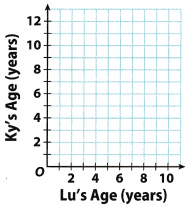Lu’s age Ky’s age 1 8 2 9 3 10 4 11 5 12

Ky’s age = Lu’s age + 7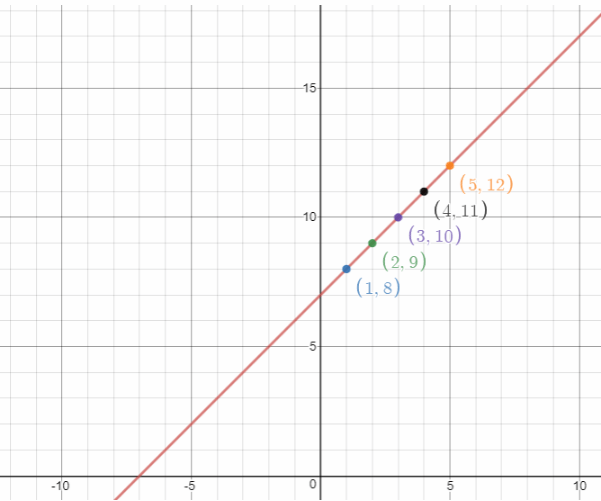Question 1.
Fred’s family already has two dogs. They adopt more dogs. Complete the table for the total number of dogs they will have. Then describe the rule. (Explore Activity)Dogs adopted Total number of dogs 1 3 2 4 3 5 4 6

Total number of dogs = Number of dogs they already have + Adopted dogs

Question 2.
Graph the relationship between the number of dogs adopted and the total number of dogs. (Example 1)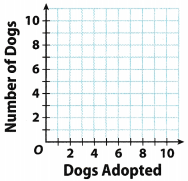List the ordered pairs from the table:
(1, 3), (2, 4), (3. 5). (4, 6)
and graph them on a coordinate plane.

Frank’s karate class meets three days every week. Complete the table for the total number of days the class meets. Then describe the rule.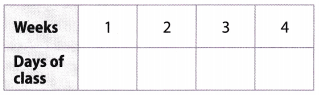Weeks Days of class 1 3 2 6 3 9 4 12

Days of class = Weeks ∙ Three days

Question 4.
Graph the relationship between the number of weeks and the number of days of class.List the ordered pairs from the table:
(1, 3), (2, 6), (3, 9), (4, 12)
and graph them on a coordinate plane.

An internet cafe charges ten cents for each page printed. Graph the relationship between the number of pages printed and the printing charge. Is the relationship additive or multiplicative? Explain.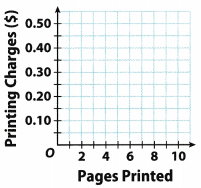Pages Printed Cost 1 10 2 20 3 30 4 40 5 50

Cost = Pages printed ∙ 10 cents
The relationship is multiplicative.

List the ordered pairs from the table:
(1, 10), (2, 20), (3, 30), (4, 40), (5, 50)
and graph them on a coordinate plane.The relationship is multiplicative.

Essential Question Check-In

Question 6.
How do you represent, describe, and compare additive and multiplicative relationships?
We use tables and ordered pairs to represent and describe additive and multiplicative relationships. You can compare these relationships by graphing them on a coordinate plane.

An additive relationship involves a constant that is added to another number.
A multiplicative relationship involves a constant that is multiplied by another number.

The tables give the price of a kayak rental from two different companies.Question 7.
Is the relationship shown in each table multiplicative or additive? Explain.
First table:
Cost = Hours ∙ 9
The relationship is multiplicative.

Second table
Cost = Hours + 40

Go Math Lesson 8.1 6th Grade Multiplicative Relationships Question 8.
Yvonne wants to rent a kayak for 7 hours. How much would this cost to each company? Which one should she choose?
First company:
Cost = Hours ∙ 9
She wants to rent it for 7 hours, so:
Cost = 7 ∙ 9 = 64

Second Company:
Cost = Hours + 40
She wants to rent it for 7 hours, so:
Cost = 7 + 40 = 47
She should choose the second company.

Question 9.
After how many hours is the cost for both kayak rental companies the same? Explain how you found your answer.
Let y represent the cast and z represents the hours.
First company:
y1 = 9x
Second company:
y2 = x + 40
If the casts are the same,
y1 = y2
Substitute y1 with 9x and y2 with x + 40:
y1 = y2
9x = x + 40
9x – x = 40
8x = 40
x = 5

Check the answer After 5 hours, in the first company the cost is:
y = 9 ∙ 5 = 45
and in the second company:
y = 5 + 40 = 45.
After 5 hours.

The graph represents the distance traveled by a car and the number of hours it takes.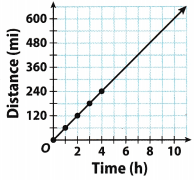Lesson 8.1 Answer Key Additive and Multiplicative Relationships Worksheets Pdf Question 10.
Persevere in Problem Solving Based on the graph, was the car traveling at a constant speed? At what speed was the car traveling?
The ordered pairs on the graph are:
(1, 60), (2, 120), (3, 180), (4, 240)
Distance = Time ∙ 60
The car was traveling at a constant speed.
Speed = $$\frac{\text { Distance }}{\text { Time }}$$ = 60 mi per hour

Constant speed; 60 mi per hour

Question 11.
Make a Prediction If the pattern shown in the graph continues, how far will the car have traveled after 6 hours? Explain how you found your answer.
Distance = Time ∙ 60
After 6 hours, distance is equal to
Distance = 6 ∙ 60 = 360
= 360 mi

Question 12.
What If? If the car had been traveling at 40 miles per hour, how would the graph be different?
Distance = Time ∙ 40

 Time Distance 1 40 2 80 3 120 4 160 5 200

List the ordered pairs from the table:
(1, 40), (2, 80), (3, 120), (4, 160), (5, 200)
and graph them on a coordinate plane.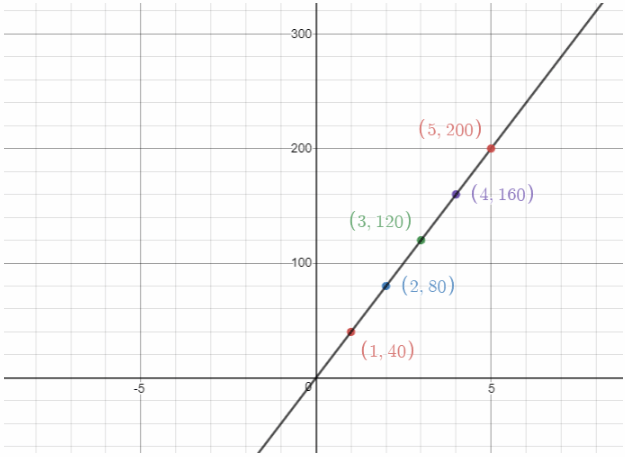Use the graph for Exercises 13 – 15.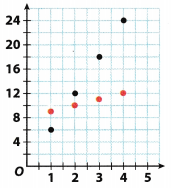Go Math Grade 6 Lesson 8.1 Answer Key Multiplicative Relationship Graph Question 13.
Which set of points represents an additive relationship? Which set of points represents a multiplicative relationship?
Ordered pairs on the graph:
(1, 6), (2, 12), (3, 18), (4, 24)
y = 6x
This set of points represents a multiplicative relationship.

(1, 9), (2, 10), (3, 11), (4. 12)
y = x + 8
This set of points represents an additive relationship.

Question 14.
Represent Real-World Problems What is a real-life relationship that might be described by the red points?
Ana is 8 years older than Ben
How old is he if she is 11 years old?

Question 15.
Represent Real-World Problems What is a real-life relationship that might be described by the black points?
In Gunther’s class there are 6 times more girls than boys.
If there are 24 girls, how many boys there are?

H.O.T. Focus On Higher Order Thinking

Question 16.
Explain the Error An elevator Tin leaves the ground floor and rises three feet per second. Lili makes the table shown to analyze the relationship. What error did she make?Distance = Time ∙ 3

 Time Distance 1 3 2 6 3 9 4 12

This is a multiplicative relationship, not an additive. If an elevator rises three feet per second, that means, in 2 seconds it will rise 3 + 3 = 2 ∙ 3 = 6 seconds, instead of 5

Question 17.
Analyze Relationships Complete each table. Show an additive relationship in the first table and a multiplicative relationship in the second table.Use two columns of each table. Which table shows equivalent ratios?
Name two ratios shown in the table that are equivalent.
First table.
B = A + 2

 A B 1 3 2 4 3 5

First table.
B = A ∙ 16

 A B 1 16 2 32 3 48

The second table shows equivalent ratios because
$$\frac{1}{16} \cdot \frac{2}{2}=\frac{2}{32}$$
$$\frac{1}{16} \cdot \frac{3}{3}=\frac{3}{48}$$

One apple pie costs $3. Find the price of 4 pies. Price = Pies ∙ 3 Additive relationship: Carl is 2 years older than his sister Julie. If she is 9 years old, how old is he? Multiplicative relationship: One apple pie costs$3. Find the price of 4 pies.